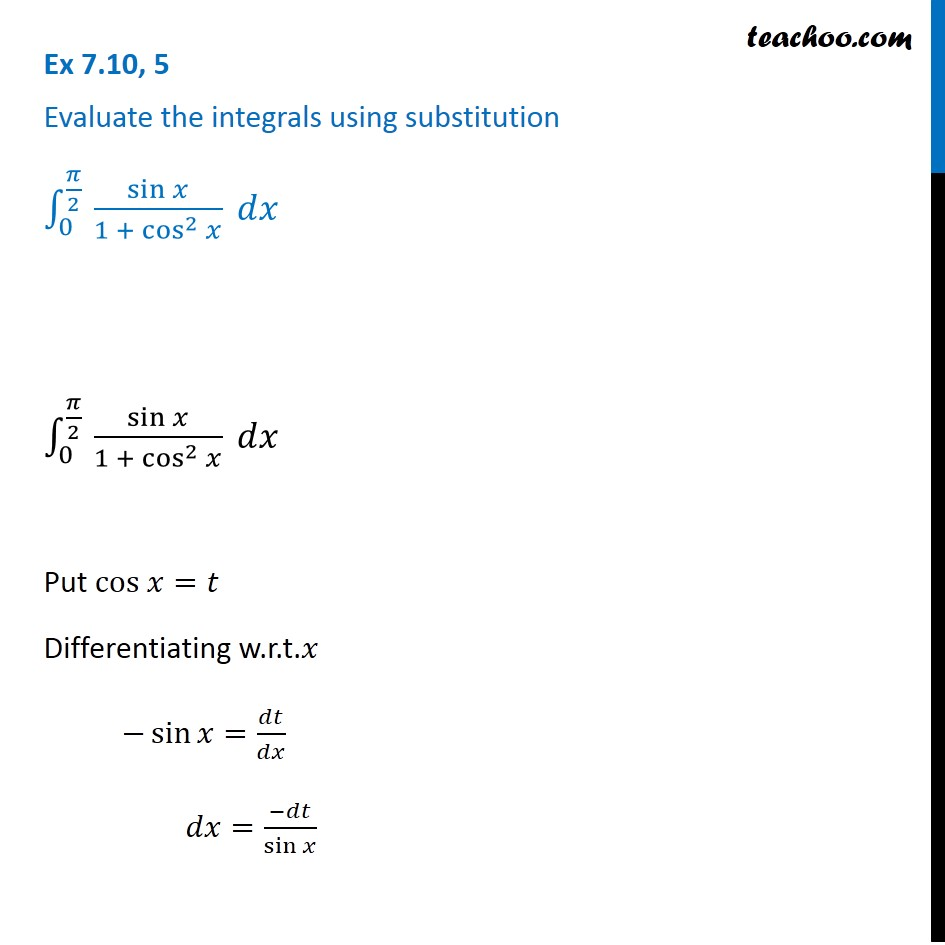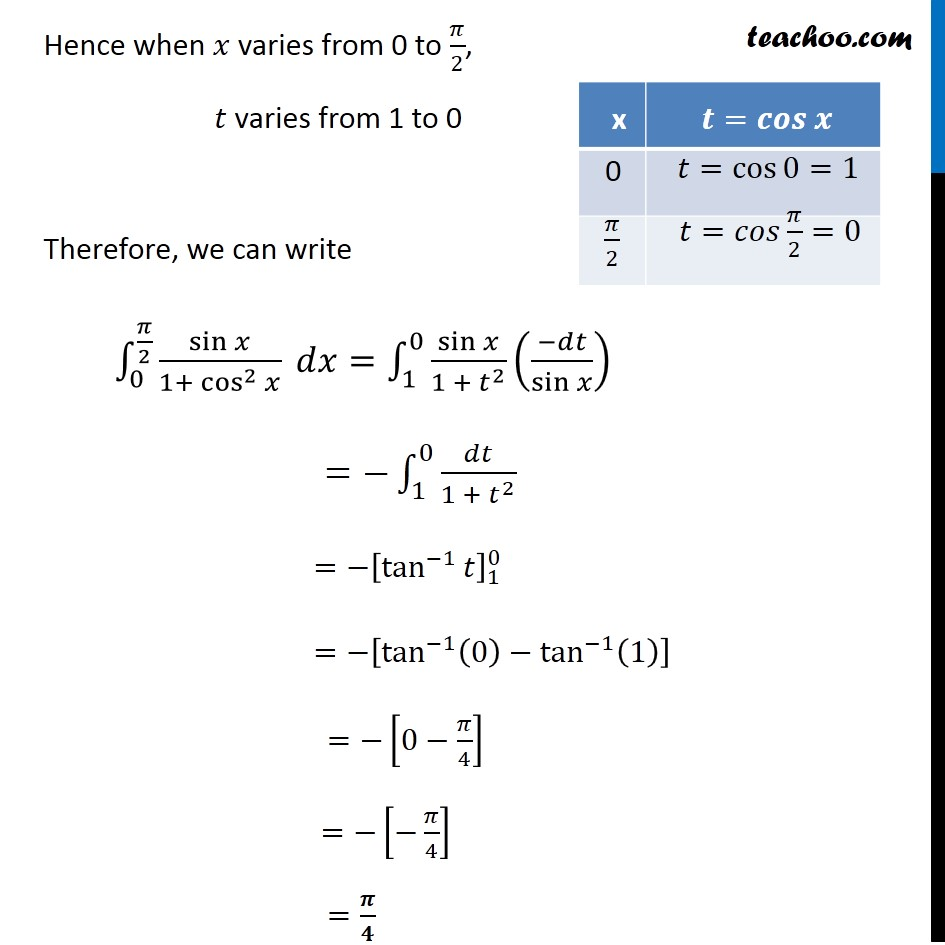Ex 7.9

Chapter 7 Class 12 Integrals
Serial order wiseLearn in your speed, with individual attention - Teachoo Maths 1-on-1 Class

### Transcript

Ex 7.9, 5 Evaluate the integrals using substitution ∫_0^(𝜋/2 )▒sin⁡𝑥/(1 + cos^2⁡𝑥 )⁡〖 𝑑𝑥〗 ∫_0^(𝜋/2 )▒sin⁡𝑥/(1 + cos^2⁡𝑥 )⁡〖 𝑑𝑥〗 Put cos 𝑥=𝑡 Differentiating w.r.t.𝑥 −sin⁡𝑥=𝑑𝑡/𝑑𝑥 𝑑𝑥=(−𝑑𝑡)/sin⁡𝑥 Hence when 𝑥 varies from 0 to 𝜋/2, 𝑡 varies from 1 to 0 Therefore, we can write ∫_0^(𝜋/2)▒sin⁡𝑥/(1+〖 cos^2〗⁡𝑥 ) 𝑑𝑥=∫_1^0▒〖sin⁡𝑥/(1 + 𝑡^2 ) ((−𝑑𝑡)/sin⁡𝑥 ) 〗 =−∫_1^0▒𝑑𝑡/(1 + 𝑡^2 ) =−[tan^(−1)⁡𝑡 ]_1^0 =−[tan^(−1)⁡〖(0)−tan^(−1)⁡(1) 〗 ] =−[0−𝜋/4] =−[−𝜋/4] =𝝅/𝟒